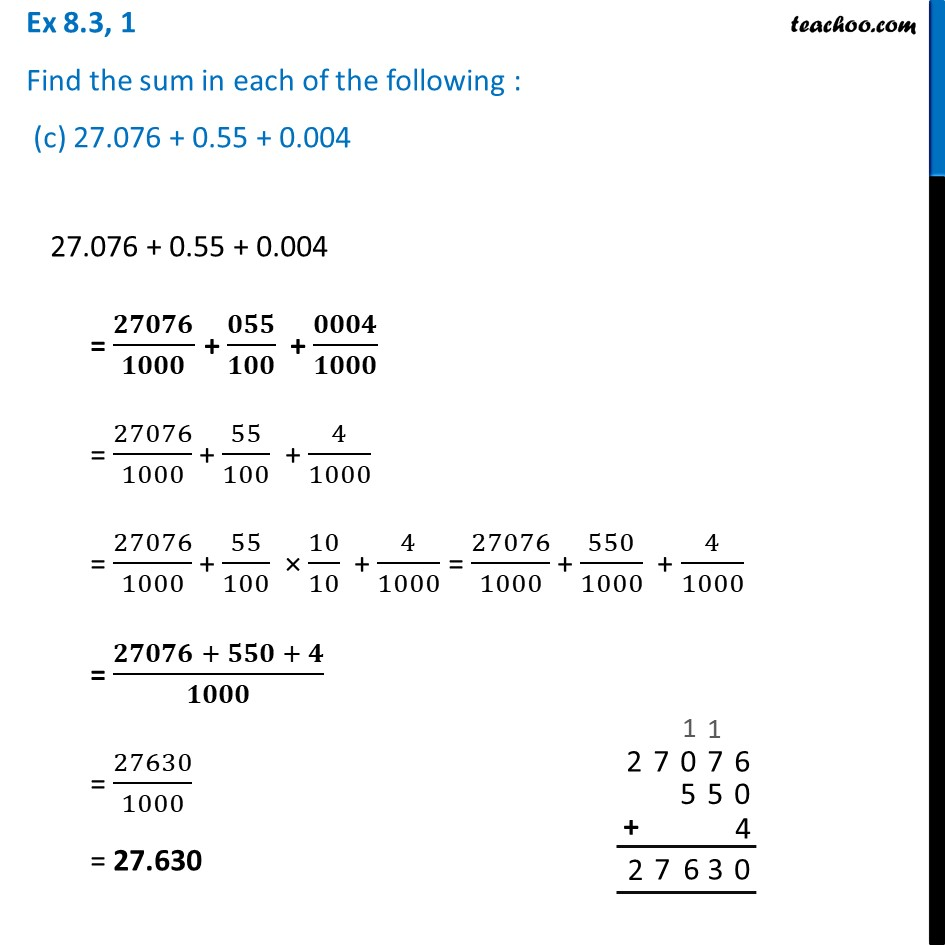Ex 8.3

Chapter 8 Class 6 Decimals
Serial order wiseLearn in your speed, with individual attention - Teachoo Maths 1-on-1 Class

### Transcript

Ex 8.3, 1 Find the sum in each of the following : (c) 27.076 + 0.55 + 0.00427.076 + 0.55 + 0.004 = 𝟐𝟕𝟎𝟕𝟔/𝟏𝟎𝟎𝟎 + 𝟎𝟓𝟓/𝟏𝟎𝟎 + 𝟎𝟎𝟎𝟒/𝟏𝟎𝟎𝟎 = 27076/1000 + 55/100 + 4/1000 = 27076/1000 + 55/100 × 10/10 + 4/1000 = 27076/1000 + 550/1000 + 4/1000 = (𝟐𝟕𝟎𝟕𝟔 + 𝟓𝟓𝟎 + 𝟒)/𝟏𝟎𝟎𝟎 = 27630/1000 = 27.630